# Solution in Presence of External Magnetic Field-Coil

Suppose that the perfectly conducting wall at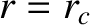is replaced by a radially-thin, magnetic field-coil that carries a helical current possessing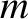periods in the poloidal direction, and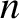periods in the toroidal direction. Let the current density in the field-coil take the form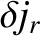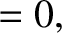(3.109)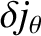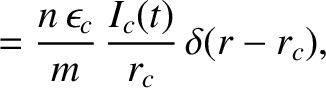(3.110)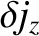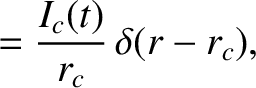(3.111)

where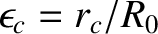. Here, the complex quantity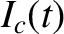specifies the amplitude and phase of the helical current flowing in the field-coil. Note that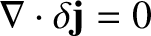, as is required by charge conservation.

The most general solution to the cylindrical tearing mode equation, (3.60), in the outer region can now be written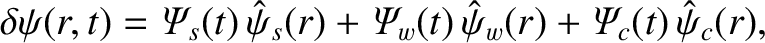(3.112)

where the real functions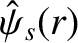and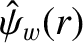are specified in Sections 3.8 and 3.9, respectively. Moreover, the real function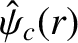is a solution of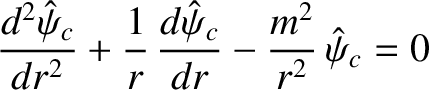(3.113)

that satisfies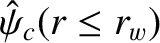(3.114)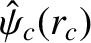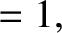(3.115)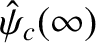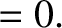(3.116)

It is easily seen that(3.117)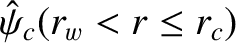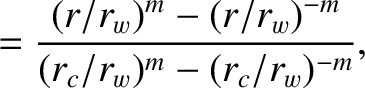(3.118)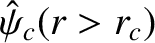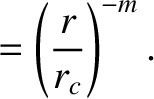(3.119)

In general,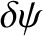is continuous across the field-coil (in accordance with Maxwell's equations), whereas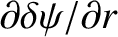is discontinuous. The discontinuity inis caused by the helical current flowing in the field-coil. The complex quantity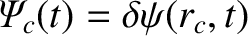(3.120)

determines the amplitude and phase of the perturbed magnetic flux at the field-coil. The complex quantity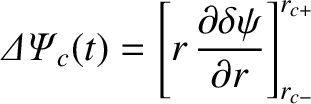(3.121)

parameterizes the amplitude and phase of the helical current sheet flowing in the field-coil. It follows from Equations (3.37), (3.38), (3.110), and (3.111) that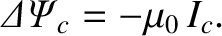(3.122)

Simultaneously matching the outer solution, (3.112), across the rational surface, the resistive wall, and the field-coil, we obtain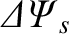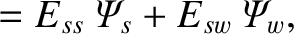(3.123)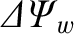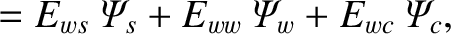(3.124)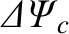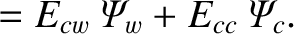(3.125)

Here,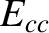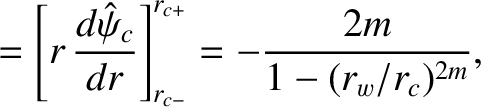(3.126)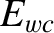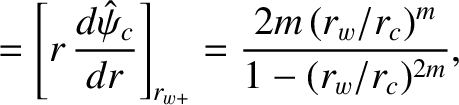(3.127)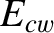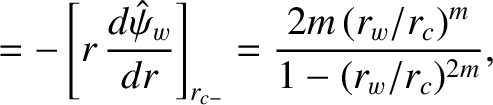(3.128)

where use has been made of Equations (3.81), (3.118), and (3.119).